Next: Discrete Approach. Up: Normal Modes and Soliton Previous: Figure 1

# Magnons on a vortex. Continuous approach.

Let's introduce small deviations of variables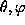from those in the vortex solution,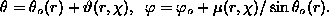In local rotated coordinate frames,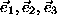, where the axis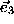coincides with the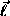-vector's direction in the unperturbed vortex, and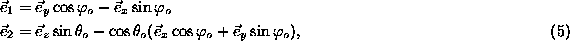the variables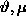are the projections of the-vector on the local axes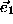and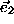: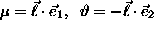.

It is easy to get the coupled set of two partial differential equations for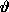and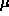: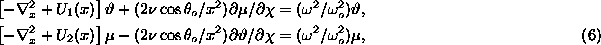where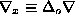, and the ``potentials'' are:\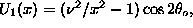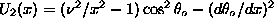. Using the ansatz like that in ,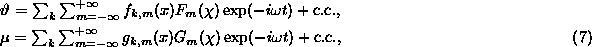where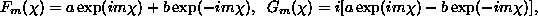and a,b are arbitrary constants, one obtains a coupled set of ODEs for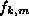and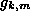. Here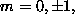...; k and m are the full set of quantum numbers denoting the eigenvalues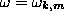The presence of the combination of exponentials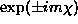with arbitrary coefficients is due to the degeneracy of the modes with m=+|m| and m=-|m|. One can see that the coupling ofandin Eq. (6) comes from the term with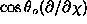only. This means that (i) the coupling vanishes exponentially at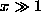(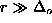); (ii) there is no coupling for m=0 modes, in contrast to the FM case ,.

Gary M Wysin
Wed Sep 6 18:51:57 CDT 1995